Скачать презентацию Area Perimeter Surface Area and Volume Review After

c587e3e81b2419dd840292ae3ec4159d.ppt

• Количество слайдов: 19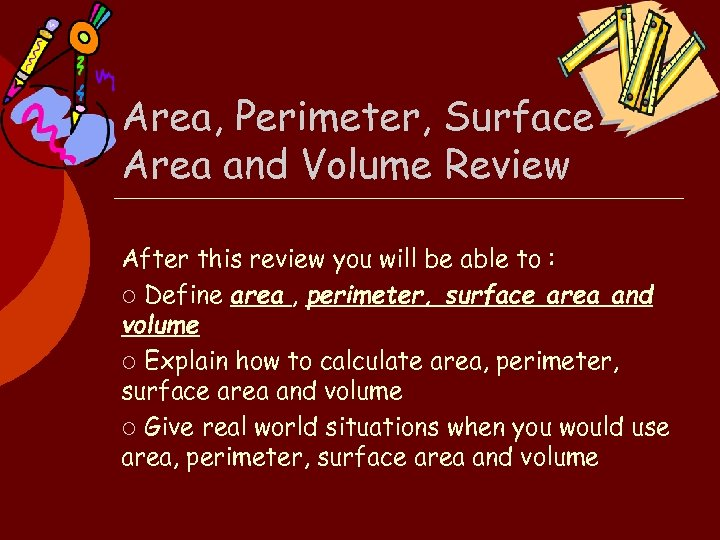Area, Perimeter, Surface Area and Volume Review After this review you will be able to : ¡ Define area , perimeter, surface area and volume ¡ Explain how to calculate area, perimeter, surface area and volume ¡ Give real world situations when you would use area, perimeter, surface area and volume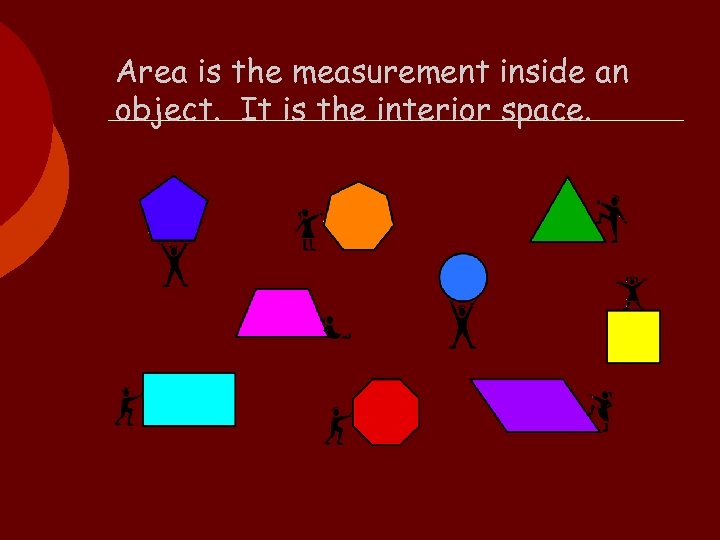Area is the measurement inside an object. It is the interior space.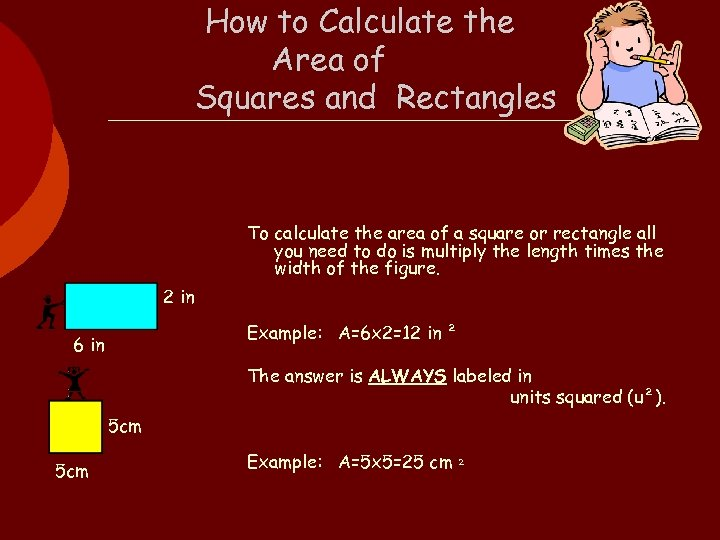How to Calculate the Area of Squares and Rectangles To calculate the area of a square or rectangle all you need to do is multiply the length times the width of the figure. 2 in Example: A=6 x 2=12 in ² 6 in The answer is ALWAYS labeled in units squared (u²). 5 cm Example: A=5 x 5=25 cm ²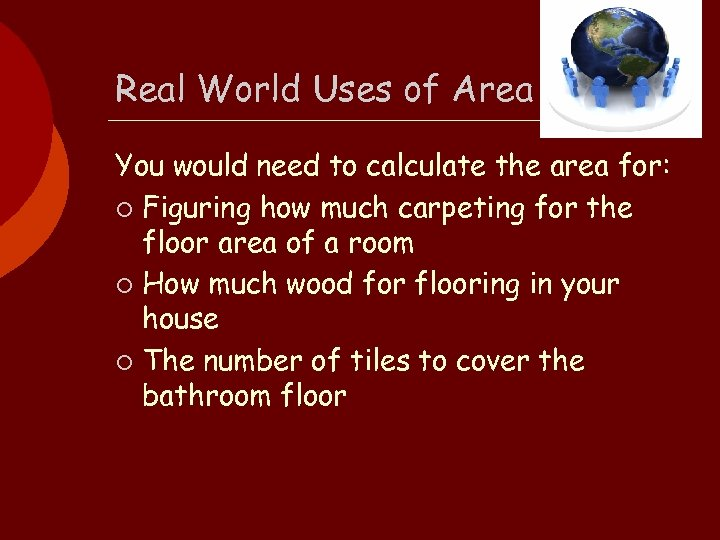Real World Uses of Area You would need to calculate the area for: ¡ Figuring how much carpeting for the floor area of a room ¡ How much wood for flooring in your house ¡ The number of tiles to cover the bathroom floor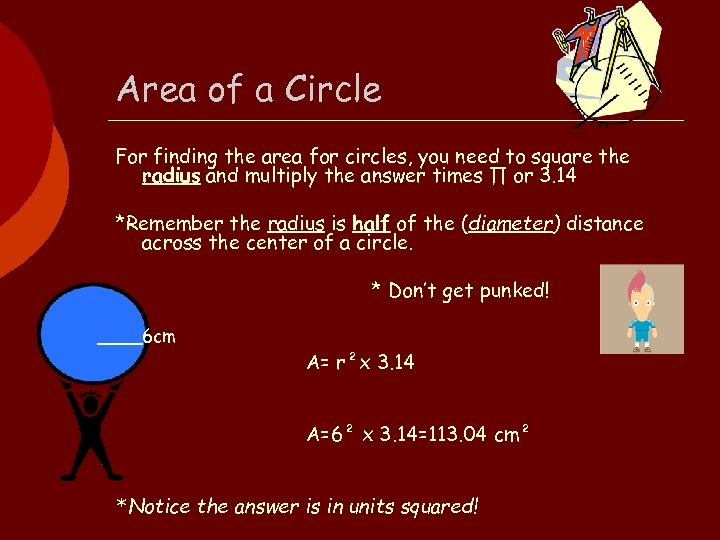Area of a Circle For finding the area for circles, you need to square the radius and multiply the answer times ∏ or 3. 14 *Remember the radius is half of the (diameter) distance across the center of a circle. * Don’t get punked! ____6 cm A= r²x 3. 14 A=6² x 3. 14=113. 04 cm² *Notice the answer is in units squared!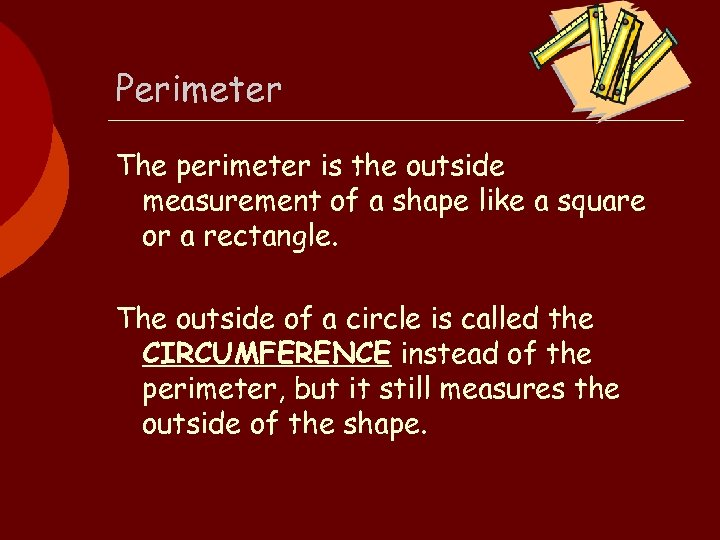Perimeter The perimeter is the outside measurement of a shape like a square or a rectangle. The outside of a circle is called the CIRCUMFERENCE instead of the perimeter, but it still measures the outside of the shape.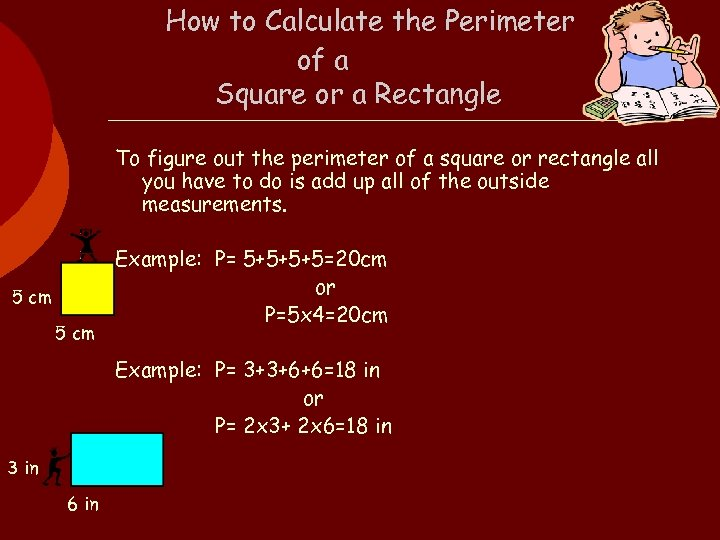How to Calculate the Perimeter of a Square or a Rectangle To figure out the perimeter of a square or rectangle all you have to do is add up all of the outside measurements. 5 cm Example: P= 5+5+5+5=20 cm or P=5 x 4=20 cm Example: P= 3+3+6+6=18 in or P= 2 x 3+ 2 x 6=18 in 3 in 6 in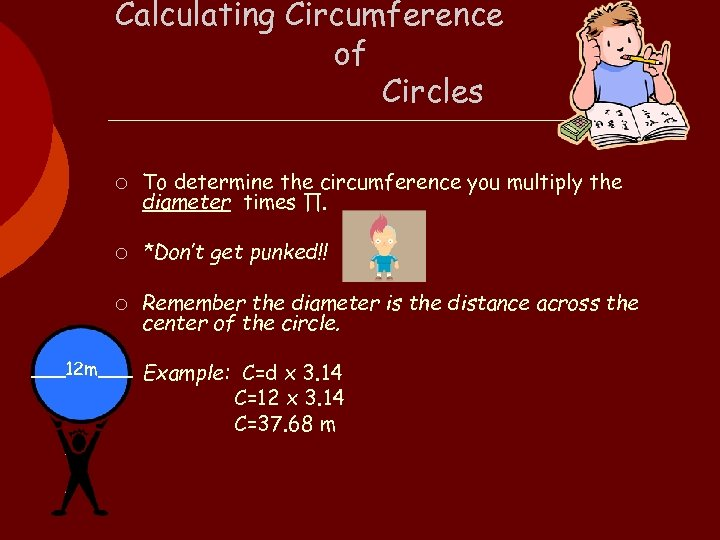Calculating Circumference of Circles ¡ To determine the circumference you multiply the diameter times ∏. ¡ *Don’t get punked!! ¡ Remember the diameter is the distance across the center of the circle. ___12 m___ Example: C=d x 3. 14 ¡ C=12 x 3. 14 C=37. 68 m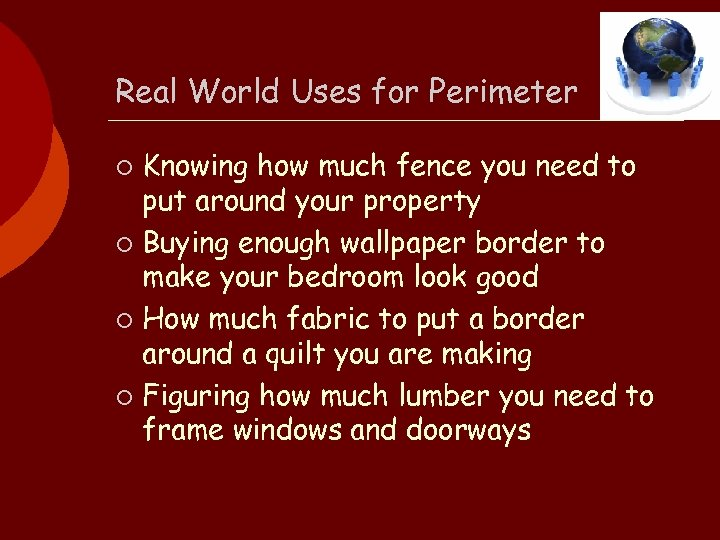Real World Uses for Perimeter Knowing how much fence you need to put around your property ¡ Buying enough wallpaper border to make your bedroom look good ¡ How much fabric to put a border around a quilt you are making ¡ Figuring how much lumber you need to frame windows and doorways ¡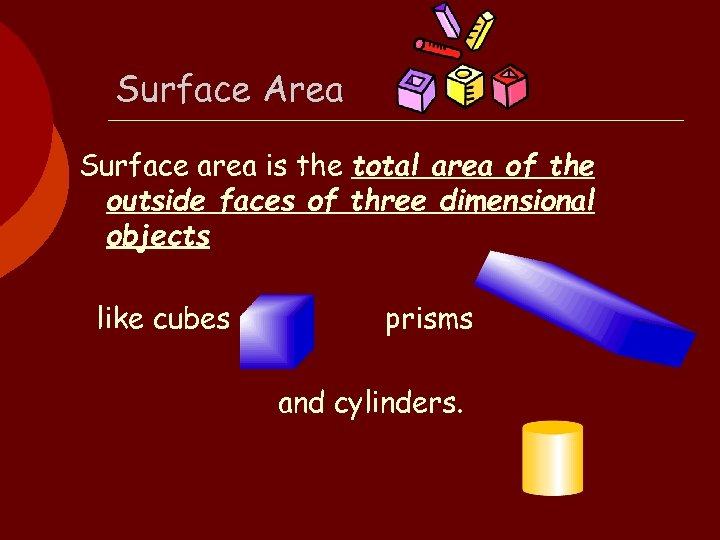Surface Area Surface area is the total area of the outside faces of three dimensional objects like cubes prisms and cylinders.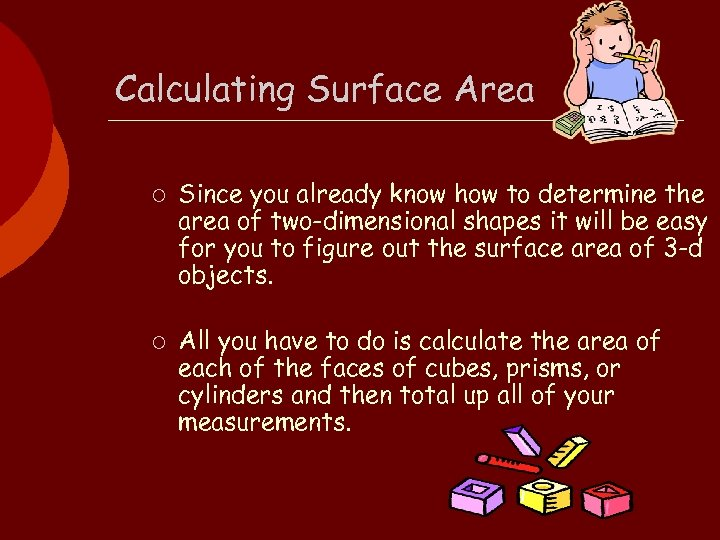Calculating Surface Area ¡ ¡ Since you already know how to determine the area of two-dimensional shapes it will be easy for you to figure out the surface area of 3 -d objects. All you have to do is calculate the area of each of the faces of cubes, prisms, or cylinders and then total up all of your measurements.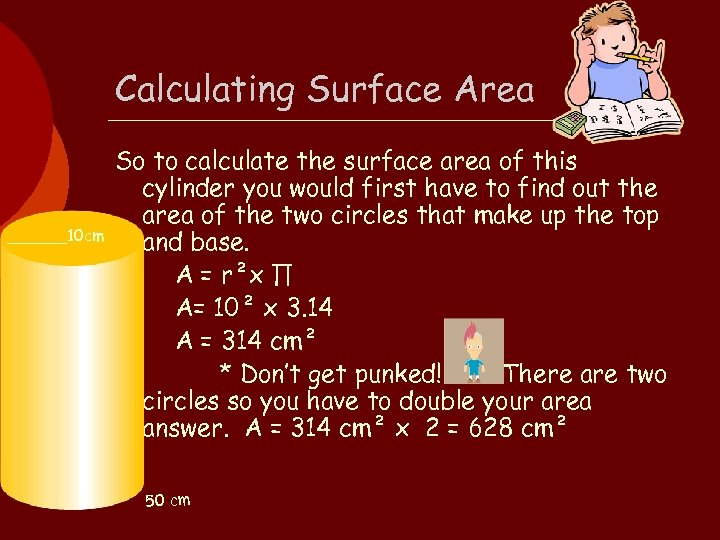Calculating Surface Area ______10 cm So to calculate the surface area of this cylinder you would first have to find out the area of the two circles that make up the top and base. A = r²x ∏ A= 10² x 3. 14 A = 314 cm² * Don’t get punked! There are two circles so you have to double your area answer. A = 314 cm² x 2 = 628 cm² 50 cm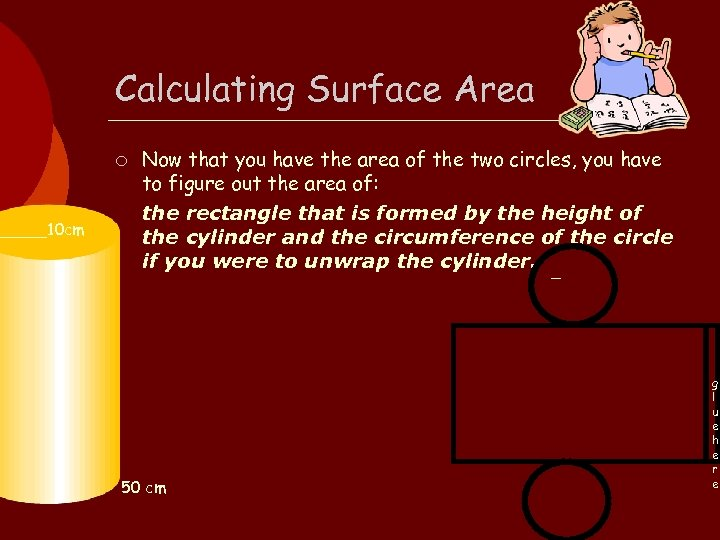_____10 cm Calculating Surface Area ¡ Now that you have the area of the two circles, you have to figure out the area of: the rectangle that is formed by the height of the cylinder and the circumference of the circle if you were to unwrap the cylinder. _ 50 cm g l u e h e r e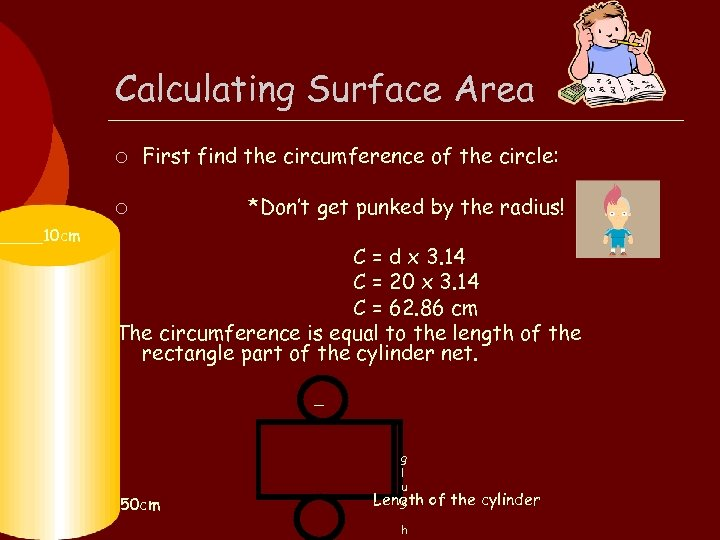_____10 cm Calculating Surface Area ¡ First find the circumference of the circle: ¡ *Don’t get punked by the radius! C = d x 3. 14 C = 20 x 3. 14 C = 62. 86 cm The circumference is equal to the length of the rectangle part of the cylinder net. _ 50 cm g l u Length e h of the cylinder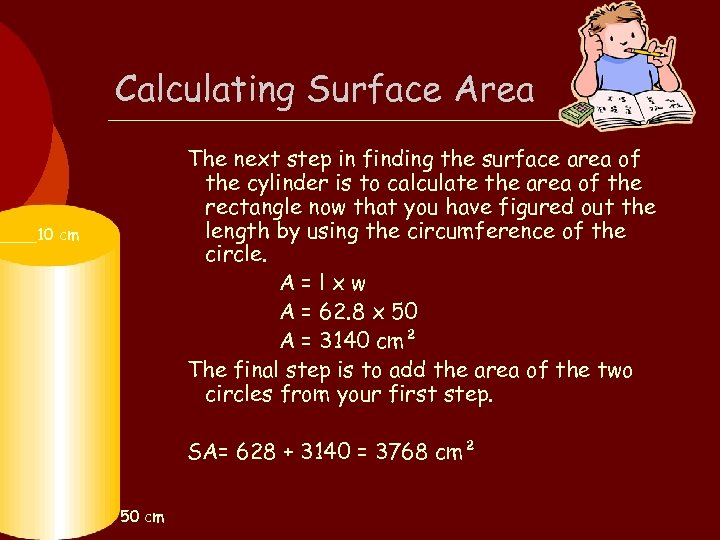Calculating Surface Area The next step in finding the surface area of the cylinder is to calculate the area of the rectangle now that you have figured out the length by using the circumference of the circle. A=lxw A = 62. 8 x 50 A = 3140 cm² The final step is to add the area of the two circles from your first step. ____10 cm SA= 628 + 3140 = 3768 cm² 50 cm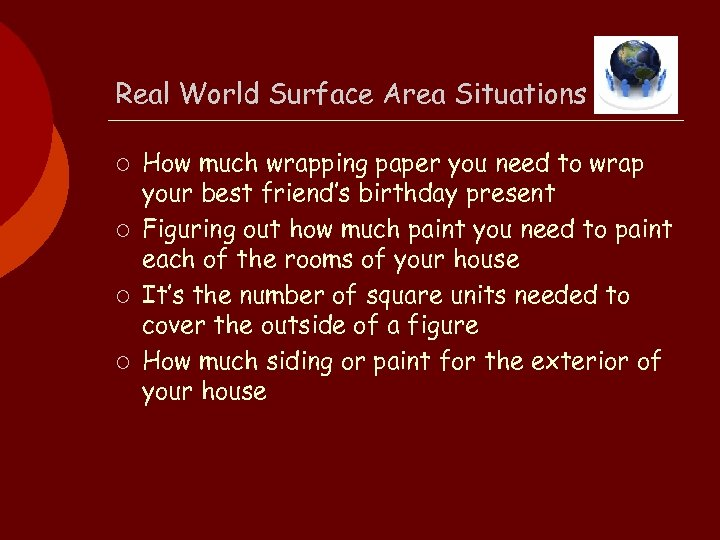Real World Surface Area Situations ¡ ¡ How much wrapping paper you need to wrap your best friend’s birthday present Figuring out how much paint you need to paint each of the rooms of your house It’s the number of square units needed to cover the outside of a figure How much siding or paint for the exterior of your house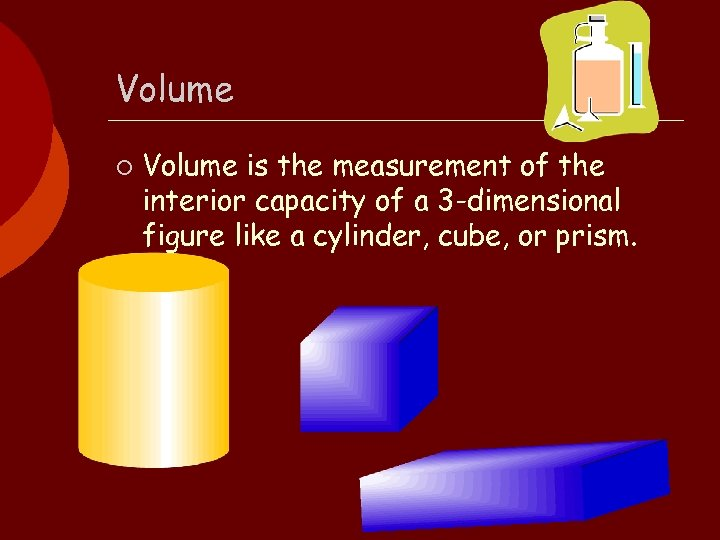Volume ¡ Volume is the measurement of the interior capacity of a 3 -dimensional figure like a cylinder, cube, or prism.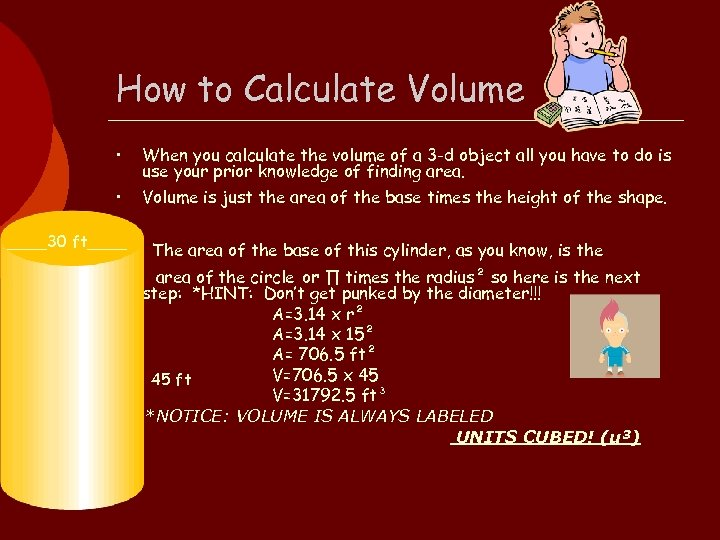How to Calculate Volume • When you calculate the volume of a 3 -d object all you have to do is use your prior knowledge of finding area. • Volume is just the area of the base times the height of the shape. ____30 ft____ • The area of the base of this cylinder, as you know, is the area of the circle or ∏ times the radius² so here is the next step: *HINT: Don’t get punked by the diameter!!! A=3. 14 x r² A=3. 14 x 15² A= 706. 5 ft² V=706. 5 x 45 45 ft V=31792. 5 ft³ *NOTICE: VOLUME IS ALWAYS LABELED UNITS CUBED! (u³)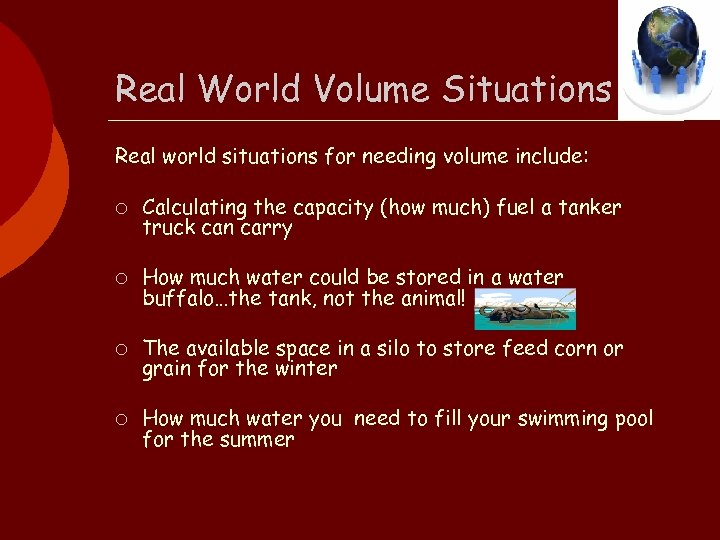Real World Volume Situations Real world situations for needing volume include: ¡ Calculating the capacity (how much) fuel a tanker truck can carry ¡ How much water could be stored in a water buffalo…the tank, not the animal! ¡ The available space in a silo to store feed corn or grain for the winter ¡ How much water you need to fill your swimming pool for the summer